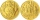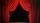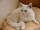7 roses

Peter buys 7 roses. When he pay for it left him 4 euro. If he bought 5 roses left him 40 euros. How many euros had Peter before buying?

Result

x =  130 eur

Solution:

x = 5(x-4)/7+40

2x = 260

x = 130

Calculated by our simple equation calculator.

Leave us a comment of example and its solution (i.e. if it is still somewhat unclear...):Be the first to comment!To solve this example are needed these knowledge from mathematics:

Do you have a linear equation or system of equations and looking for its solution? Or do you have quadratic equation?

Next similar examples:

1. CakesGrandmother baked cakes. Half of its was poppy, quarter with plum jam and 16 cheesecakes. How many cakes she baked in total?
2. Far countryIn a country far away, the value of 3 pesos is 12 centavos more than the value of 1 peso. How many centavos is 1 peso worth?
3. NormThree workers planted 3555 seedlings of tomatoes in one dey. First worked at the standard norm, the second planted 120 seedlings more and the third 135 seedlings more than the first worker. How many seedlings were standard norm?
4. PerformanceSame number of tickets sold for 15 CZK, 20 CZK, 30 CZK and 40 CZK a total of CZK 13,230 on theatre performance. How many tickets were sold for this performance?
5. Forest nurseryIn the forest nursery after winter, they found that 1/10 stems died out of them. For them, they land 193 new spruces. How many spruces are in the forest nursery?
6. Money 6Lita had some money. She spent 1/2 of it on a hand bag and 1/3 of the remainder of the blouse. She had 260.00. How much money did she have at start?
7. Simple equationSolve the following simple equation: 2. (4x + 3) = 2-5. (1-x)
8. Find xSolve: if 2(x-1)=14, then x= (solve an equation with one unknown)
9. EquationSolve the equation: 1/2-2/8 = 1/10; Write the result as a decimal number.
10. One-thirdA one-third of unknown number is equal to five times as great as the difference of the same unknown number and number 28. Determine the unknown number.
11. CatOne-fifth of the monthly pocket money contributes Maros for food for his cat, half of the rest postpone for a new smartphone. The remaining € 8 spend. How much pocket money gets Maros a month?
12. Unknown numberIdentify unknown number which 1/5 is 40 greater than one tenth of that number.
13. Simple equationSolve for x: 3(x + 2) = x - 18
14. Unknown number 11That number increased by three equals three times itself?
15. Equation?
16. Equation 29Solve next equation: 2 ( 2x + 3 ) = 8 ( 1 - x) -5 ( x -2 )
17. Negative in equation2x + 3 + 7x = – 24, what is the value of x?